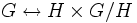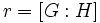Lagrange's theorem

This article gives the statement, and possibly proof, of a basic fact in group theory.
View a complete list of basic facts in group theory
VIEW FACTS USING THIS: directly | directly or indirectly, upto two steps | directly or indirectly, upto three steps|
This article states a result of the form that one natural number divides another. Specifically, the (order of a group) of a/an/the (subgroup) divides the (order of a group) of a/an/the (group).
View other divisor relations |View congruence conditions
This article states a result of the form that one natural number divides another. Specifically, the (size) of a/an/the (left coset space) divides the (order of a group) of a/an/the (group).
View other divisor relations |View congruence conditions

Statement

Verbal statement

In a finite group, the order of any subgroup divides the order of the group. In fact, the ratio of the orders is the index of the subgroup, which is the same as the number of left cosets, and as the number of right cosets, of the subgroup.

Statement with symbols

Let$G$ be a finite group, and$H$ be a subgroup. Then, if$|G|, |H|$ denote the orders of these groups (i.e., the cardinalities of their underlying sets) and$[G:H]$ denotes the index of$H$ in$G$, then:$|G| = |H|[G:H]$

Related facts

Stronger facts

• Index is multiplicative: This states that if$K \le H \le G$ are subgroups then$[G:K] = [G:H][H:K]$. Lagrange's theorem is a special case with$K$ the trivial subgroup.

Applications

Lagrange's theorem is extremely useful in providing information about the size and structure of subgroups given information about the size of the whole group. Here are some obvious corollaries of Lagrange's theorem:

• Subgroup of size more than half is whole group: This turns out to be useful when trying to prove that a certain subgroup is the whole group. It is also used to design probabilistic tests of primality.
• Prime order implies no proper nontrivial subgroup
• Order of element divides order of group: In a finite group, the order of any element, i.e., the smallest positive integer$n$ for which its$n^{th}$ power is the identity element, is a divisor of the order of the group.

Normal subgroups and surjective homomorphisms

Combining Lagrange's theorem with the first isomorphism theorem, we see that given any surjective homomorphism$\varphi:G \to H$ of finite groups$G$ and$H$, the order of$H$ must divide the order of$G$. That's because, if$N$ is the kernel of the homomorphism, the first isomorphism theorem identifies$H$ with the quotient group$G/N$, whose order equals the index$[G:N]$. See:

In fact, more is true: the surjective homomorphism from$G$ to$H$ has the property that the inverse images of all elements of$H$ have equal size. Further information: Variety of groups is congruence-uniform

Transitive group actions

Further information: orbit-counting theorem, fundamental theorem of group actions

If$G$ acts transitively on a set$S$, and$H$ denotes the isotropy subgroup of a point$s \in S$, then the set$S$ can be identified with the left coset space$G/H$. In particular, we get:$|G| = |H| |S|$

Hence, the order of$S$ divides the order of$G$.

Converse

Further information: Group having subgroups of every order dividing the group order

While there's no direct formulation of a converse to Lagrange's theorem, one plausible converse might be: given any finite group$G$, and any positive divisor$d$ of the order of$G$, there exists a subgroup$H$ of$G$ of order$d$. This is, however false. The smallest counterexample is the alternating group on four elements, which has order 12 and has no subgroup of order 6 (see its subgroup structure). However, partial versions of the converse are true:

1. For a cyclic group, there is a unique subgroup of every order dividing the order of the group.
2. A group of prime power order, and more generally, a finite nilpotent group, and even more generally a finite supersolvable group, has subgroups of every possible order dividing the order of the group. For full proof, refer: Prime power order implies subgroups of all orders dividing the group order, Finite supersolvable implies subgroups of all orders dividing the group order
3. Sylow's theorem: In any finite group, there exist Sylow subgroups. A Sylow subgroup is a group of prime power order, whose order and index are relatively prime.
4. Hall's theorem: A finite group is a finite solvable group if and only if it has Hall subgroups of all permissible orders. A Hall subgroup is a subgroup whose order and index are relatively prime.
5. Every finite solvable group is a subgroup of a finite group having subgroups of all orders dividing the group order

Choice of coset representatives

Lagrange's theorem proves that the order of a group equals the product of the order of the subgroup and the number of left cosets. There is, however, no natural bijection between the group and the Cartesian product of the subgroup and the left coset space. In other words, for a subgroup$H \le G$, there is no natural bijection:$G \leftrightarrow H \times G/H$.

We can obtain a bijection if we choose a set of coset representatives, i.e., we make a choice of one element in each left coset. Such a choice gives a specific bijection between the subgroup and each of its left cosets. Essentially, the coset representative is used as a reference point. To describe an element of$G$, we describe its coset, and the ratio between that element and its coset representative, and this yields the bijection.

However, there is in general no natural choice of coset representatives, and being able to choose coset representatives requires the axiom of choice. The Vitali construction of a non-measurable set, as well as the Banach-Tarski paradox, use precisely this ability.

Infinite versions

Further information: Cardinal multiplication#Lagrange's theorem

While Lagrange's theorem is typically stated for a finite group, the reasoning used in its proof also works for infinite groups. Thus, if$G$ is any group,$H$ is a subgroup, then, in the sense of cardinals:$|G| = |H|[G:H]$

The theory of multiplication of infinite cardinals allows us to determine the cardinality of$G$ knowing the cardinality of$H$ and$[G:H]$; however, it does not permit us to always compute successfully the cardinality of$H$ using the other two, or to compute the index$[G:H]$ using the other two quantities.

Analogues

Groups with measures

Lagrange's theorem has analogues in groups equipped with measures (in fact, the analogues make sense in groups equipped with finitely additive functions, such as amenable discrete groups). Here, we replace the order of the group and subgroup by their measures, while the index remains the index. Thus, if$G$ is a group with a left-invariant measure$m$ and$H$ is a measurable subgroup of$G$, of finite index in$G$, then:$\! m(G) = m(H)[G:H]$

Further information: Lagrange's theorem for measures

Weaker algebraic structures

The analogue of Lagrange's theorem fails to hold for structures like magmas, semigroups, and monoids. There are two problems: the left cosets of any submonoid are not necessarily pairwise disjoint, and the left cosets may not have equal size. For a cancellative monoid, the left cosets do always have equal size, but they still may not be pairwise disjoint.

If we work with loops, then the chief problem is the lack of associativity. Given a subloop of a loop, it is possible to talk of its left cosets, and they all have the same size, but due to the absence of associativity, we cannot guarantee that the left cosets are pairwise disjoint. However, it turns out that if we impose somewhat restrictive associativity assumptions, then certain subgroups inside loops do have pairwise disjoint left cosets, and Lagrange's theorem gives some information. An example is the octonion loop.

More generally, we have the following terminology:

Stronger algebraic structures

Lagrange's theorem works for any variety of algebras that admits the variety of groups as a reduct. In other words, it works for algebraic structures stronger than groups. Hence, we have:

1. The order of any subring of a finite ring divides the order of the ring.
2. The order of any submodule of a finite module over a ring divides the order of the module.

For subfields of rings, there is an even stronger relationship than being a divisor: the order of a ring must be a power of the order of any subfield of the ring. That's because the ring acquires the structure of a vector space over the field, and we can choose a basis for this vector space.

Facts used

1. Left cosets partition a group: So the group is a disjoint union of left cosets of the subgroup
2. Left cosets are in bijection via left multiplication: All the left cosets have the same size.

Proof

Given: A finite group$G$, a subgroup$H$

To prove:$|G| = |H|[G:H]$

Proof: From fact (1), we know that we can write$G$ as a disjoint union of left cosets of$H$. Let$r = [G:H]$ be the number of left cosets of$H$ and$A_1,A_2, \dots, A_r$ be the left cosets of$H$. Then, we can write:$G = \bigsqcup_{i=1}^r A_i$

(the$\sqcup$ symbol stands for disjoint union).

Taking cardinalities both sides:$|G| = \sum_{i=1}^r |A_i|$

By fact (2)$|A_i| = |H|$ for each left coset$A_i$ of$H$, so:$|G| = \sum_{i=1}^r |H| = r|H| = |H|[G:H]$